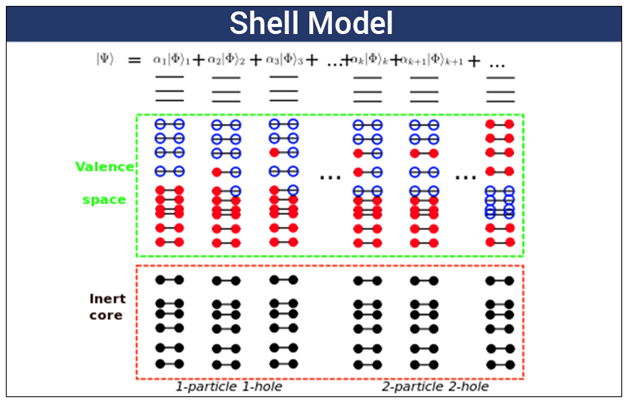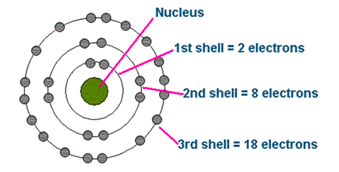Checkout JEE MAINS 2022 Question Paper Analysis : Checkout JEE MAINS 2022 Question Paper Analysis :

# Shell Model

The nuclear shell model is a model of the atomic nucleus. It uses the Pauli exclusion principle to explain the nucleus structure in terms of energy levels. In order to study the complete nucleus structure, various nuclear shell models were proposed. The first shell model was introduced in the year 1932 by Dmitry Ivanenko and later on developed by various physicists – Maria Goeppert Mayer, Eugene Paul Wigner, and J.Hans.D. Jensen in the year 1949.

## Nuclear Shell Model

It basically explains the distribution of energy levels into different atom shells and nucleus atom shells. A shell is described as the energy level where particles having the same energy exist. In this model, all the nuclear particles are paired one-to-one, neutron with a neutron, and proton with a proton. The paired neutrons and protons in nuclear energy levels are filled when the number of neutrons or protons is equal to 2, 8,20,28,50,82, or 126. These are the magic numbers that show the most stable nuclei.The unpaired ones are responsible for the properties of a nucleus and valence electrons are responsible for different chemical properties of elements. With the help of the shell model, we can accurately predict the properties of nuclei such as angular momentum. But, for nuclei which are in a highly unstable state, the shell model needs to be modified or replaced with other models such as the collective model, liquid-drop model, and compound nucleus model.

## Shell Model of the Atom

It explains the arrangement of different electrons present around the nucleus of an atom as per energy levels.Shell Model of the Atom

 Atomic Number = number of electrons in an atom

The atomic shell model explains the structure of atoms. The negatively charged fundamental particles which are known as electrons are considered to occupy diffuse shells in the space that surrounds the positively charged nucleus. The shell which is closest to the nucleus is the first shell.

The shells are designated as:

 K First shell L Second shell M Third shell and so on

The sequence in which the electrons occupy the shell is given as:

2, 8, 18, 32, 50, 72, 98.

The atomic shell model also helps in understanding the chemical properties of the atoms.

The maximum number of electrons that can occupy the energy level in an atom is found by using the following formula:

 Electron capacity = 2n2

Where n is the principal quantum number.

Following is the table for the shell levels and their electron capacity:

 Energy Level Shell Designation Maximum no.of electrons 1 K 2 2 L 8 3 M 18 4 N 32 5 O 50 6 P 72

### Difference Between Shell Structure of Nuclei and Shell Structure of Atom

 Shell Structure of Nuclei Shell Structure of Atom Energy Level starts from Zero state Energy Level starts from the first state Magic Numbers,  Z= 2,8,20,28,50,82,126 and so on Magic Numbers, Z = 2,10,18,36,54,86 and so on The average potential of Nuclei is different from that of an atom The average Potential varies. Particles of the shell structure of nuclei are neutrons and protons Particles of the shell structure of an atom are electrons

### Magic Numbers in Nuclear Structure

When a nucleus has an even number of protons and neutrons, such a nucleus is more stable than with the odd numbers. This number is known as “magic numbers” and they offer stability to the atom. Following is the sequence of magic number:

2, 8, 20, 28, 50, 82, 126.

When nuclei will have both neutron number and proton number equal to one of the magic numbers, they are known as “doubly magic”. Calcium is an example of a nucleus that has a doubly magic number.

## Frequently Asked Questions – FAQs

### What is the formula to calculate the electron capacity?

Electron capacity = 2n2

### How many electrons can M shells hold?

M shell can hold 18 electrons.

### Give an example of a compound which has a doubly magic number nucleus?

Calcium is an example of a nucleus that has a doubly magic number.

### What does the shell model number of atoms explain?

Shell model number of atoms explains the arrangement of different electrons present around the nucleus of an atom as per energy levels.

### Which term explains the number of electrons in an atom?

Number of electrons in an atom is given by atomic number.

Stay tuned with BYJU’S to learn more related physics concepts with the help of engaging video lessons.

Test your knowledge on Shell Model